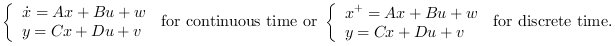Scilab 6.0.1
Change language to:
English - Français - 日本語 - Português -

See the recommended documentation of this function

Справка Scilab >> CACSD > Control Design > Linear Quadratic > lqg2stan

# lqg2stan

LQG to standard problem

### Syntax

`[P_aug,r]=lqg2stan(P,Qxu,Qwv)`

### Arguments

P22

State space representation of the nominal plant (`nu` inputs, `ny` outputs, `nx` states).

Qxu

`[Q,S;S',N]` symmetric `nx+nu` by `nx+nu` weighting matrix.

Qwv

`[R,T;T',V]` symmetric `nx+ny` by `nx+ny` covariance matrix.

r

Row vector `[ny nu]`.

P_aug

Augmented plant state space representation (see: syslin)

### Description

`lqg2stan` returns the augmented plant for linear LQG (H2) controller design problem defined by:

• The nominal plant `P22`:described by• The (instantaneous) cost function.

• The noises covariance matrixUp to Scilab-5.5.2 lqg2stan returns wrong inverted values (see bug 13751) to obtain the good result one had to use `[P,r]=lqg2stan(-P,Qxu,Qwv)`. This bug is fixed since Scilab-6.0.0, old codes must be modified accordingly.

### Algorithm

If `[B1;D21]` is a factor of `Qxu`, `[C1,D12]` is a factor of `Qwv` (see: fullrf) then `P_aug=syslin(P.dt,P.A,[B1,P.B],[C1;-P.C],[0,D12;D21,P.D])`

### Examples

```ny=2;nu=3;nx=4;
P22=ssrand(ny,nu,nx);
Qxu=rand(nx+nu,nx+nu);Qxu=Qxu*Qxu';
Qwv=rand(nx+ny,nx+ny);Qwv=Qwv*Qwv';
[P_aug,r]=lqg2stan(P,Qxu,Qwv);
K=lqg(P_aug,r);  //K=LQG-controller
spec(h_cl(P_aug,r,K))      //Closed loop should be stable
//Same as Cl=P22/.K; spec(Cl('A'))

s=poly(0,'s')
lqg2stan(1/(s+2),eye(2,2),eye(2,2))```